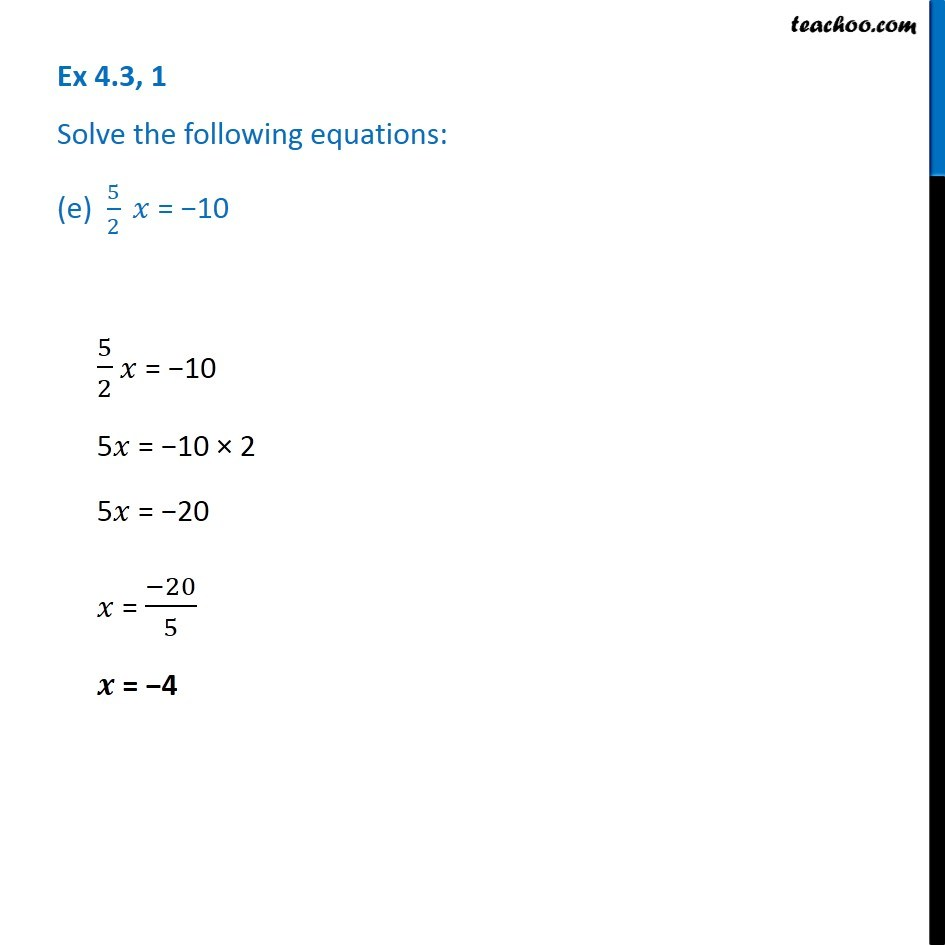1. Chapter 4 Class 7 Simple Equations
2. Serial order wise
3. Ex 4.3

Transcript

Ex 4.3, 1 Solve the following equations: (e) 5/2 𝑥 = −105/2 𝑥 = −10 5𝑥 = −10 × 2 5𝑥 = −20 𝑥 = (−20)/5 𝒙 = −4